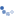# Mz moment due to tendon in curved structure

Question:

Hello,

I have a problem with a two-span continuous beam that has horizontal curve. The span lengths are 2x30m.
I defined the tendon with stressing at both the ends. I purposefully route the cable in the center of the cross section without any eccentrics, so that the results are not affected by internal eccentricity.

So, on stressing the tendon, the bridge should try to straighten and I should get moment diagram in Mz like how I would get in My for self weight. However, when I check the Mz moment due to tendon primary after stressing, it isn't as I expected. Its like each element is behaving as a simply supported beam for Mz moment! Why is it happening? What am I doing wrong?

Before proceeding to the answer, lets just clarify on how the tenodn is working right now. Tendon primary is nothing but Force in tendon multiplied by eccentricity of the tendon with the element. Now, lets just consider a single element for our example.
1) Using the Curve Option:Mz moment due to tendon primary = Force in tendon x eccentricity as shown below.Image above shows a magnified view of the element and how a tendon would be considered for calculating the forces when the curve option is selected. The moment that you get, its like simply supported beam between 2 nodes, because the eccentricity would be 0 at nodes and maximum at center of element in transverse direction. Also, it was noted that R was entered as 80 for all tendon coordinates. That isn't necessary. Radius of curvature is take automatically.
Hence, if we further divide the elements, it could be noted that this moment reduces, though it still isn't 0.Practically speaking, this moment should be 0 since my tendon and element are concentric. This can be observed in Round Option (Fine) model. Hence, we talk about the next option.
2) Using the Straight option:In this case, the tendon is taken as straight between the 2 entered coordinates. Hence, the tendon the the element would be exactly concentric as the case actually is and the tendon primary moment would be absolutely 0. This could be noted from the Straight Option attached with this response.
About the expected moment, I believe that is actually tendon secondary and not tendon primary. Kindly check the tendon secondary Mz for the Straight Option model.Personally, I'd recommend using straight rather than curve, because that has similar approximation for tendon and element, as it would practically be. Kindly let me know if further assistance would be required.

Regards,
Nandeep
Technical Manager, MIDAS
Creation date: 6/13/2018 6:22 AM (Nandeep Gohil)      Updated: 8/29/2018 11:03 AM (dklee@midasit.com)